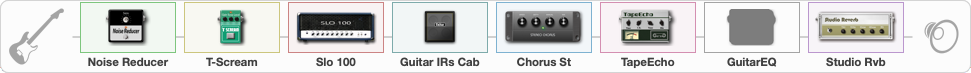# 1980's Metal Guitar Solo

Discussion in 'ToneLib-GFX presets' started by davidtaylors, Apr 19, 2020.

1. ### davidtaylorsWell-Known Member

1980's Metal Guitar Solo

Preset name: 80's Metal Guitar Solo

Made for every 80's guitar solo

Effects chain:Effect: "Noise Reducer" (Dynamics / Filter), active - "yes"
{
"Sens" = 71
"Mode" = Hard
}

Effect: "T-Scream" (Overdrive / Distortion), active - "yes"
{
"Drive" = 74
"Tone" = 39
"Level" = 99
}

Effect: "Slo 100" (Amp simulators), active - "yes"
{
"Gain" = 66
"Bass" = 51
"Middle" = 21
"Treble" = 77
"Presence" = 33
"Master" = 65
"Level (dB)" = 0
}

Effect: "Guitar IRs Cab" (Cabinets), active - "yes"
{
"Model" = Peavey 5150 (4x12")
"Mic Position" = Edge
"Mic Distance" = Middle
"Low Cut (Hz)" = 73
"Hi Cut (kHz)" = 20.0
"Mix" = 100
"Level (dB)" = 3
}

Effect: "Chorus St" (Modulation / Sfx), active - "yes"
{
"Speed" = 2.8
"Depth" = 58
"Center" = 6.1
"Mix" = 65
}

Effect: "TapeEcho" (Delay), active - "yes"
{
"Time" = 316
"Feedback" = 38
"Tone" = 65
"LoDamp" = 19
"Mix" = 65
}

Effect: "GuitarEQ" (Dynamics / Filter), active - "no"
{
"160 Hz" = 5
"400 Hz" = 6
"800 Hz" = 5
"1.6 kHz" = 4
"3.2 kHz" = 4
"6.4 kHz" = 5
"12 kHz" = 0
"Level (dB)" = 0
}

Effect: "Studio Rvb" (Reverb), active - "yes"
{
"Time" = 8.7
"PreDelay" = 32
"LoDamp" = 100
"HiDamp" = 22
"Mix" = 62
}

Note: You will need to download and install the ToneLib-GFX software to use the preset.

File size:
789 bytes
Views:
9,158
2. Very cool!